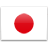S Q T L

S Q T L

S Q L T

S Q L T

S Q L T

S L Q T

S L Q T

S Q L T

S Q L T

S Q L T

S Q T L

S Q L T

S L Q T

S L Q T

S L Q T

S Q L T

S L Q T

S L Q T

S L Q T

S Q L T

Q L T S

S L Q T

S L T Q

S L T Q

S L Q T

S L T

S L T Q

S L T Q

S L T Q

S L T

S L T Q

Q T L S

Q T S L

T Q S

L T S

T Q L

L T S

T L S

S Q T L

T L S

T L S

T L S

T S L